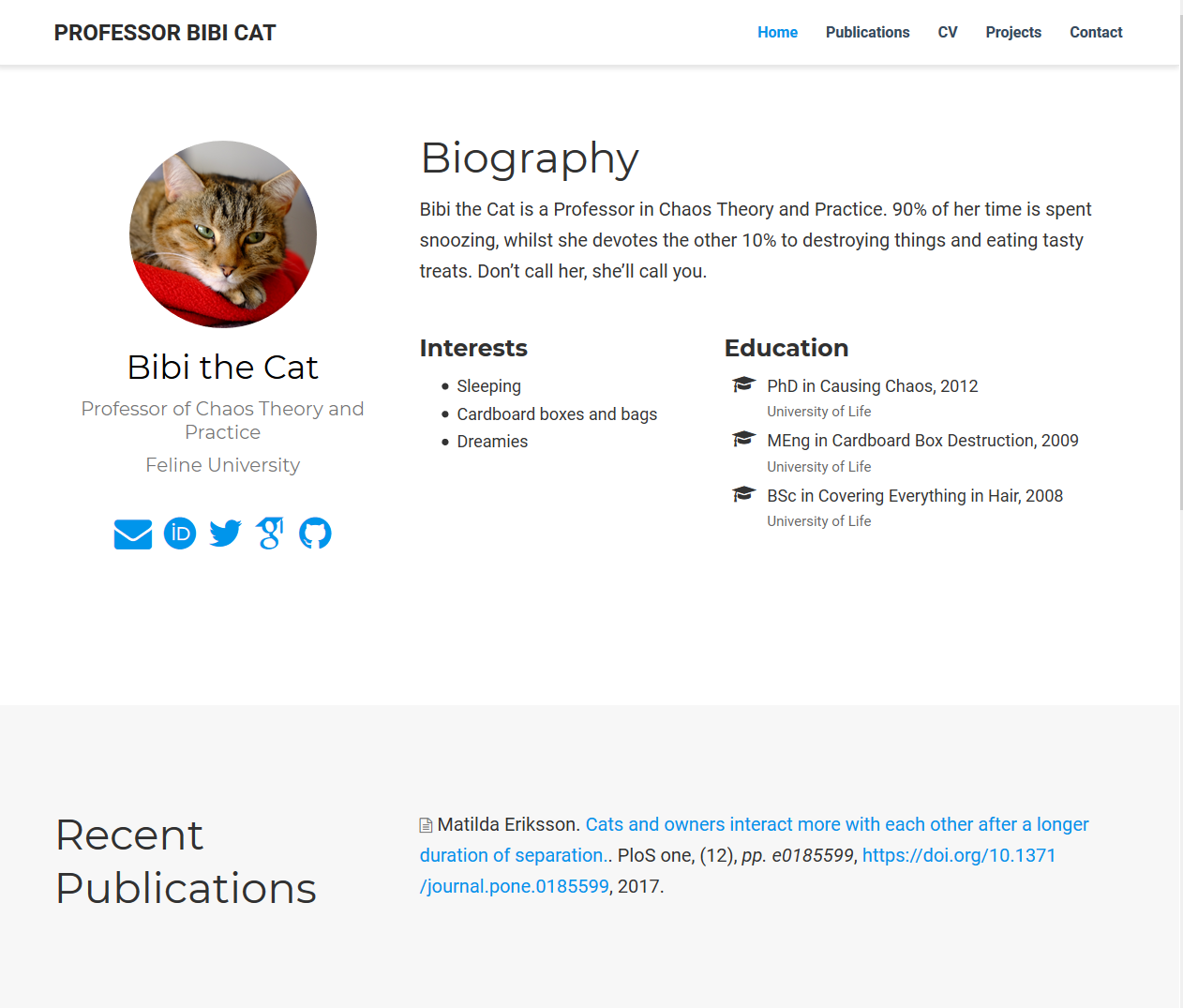# 4 Creating publication files

This is probably the most fiddly part. In the `content/publication` folder we need `.md` files for each publication we want to add to our site.

Bibi being quite a lazy cat only has one publication, but we’ll look at how to automate the process for many.

## 4.1 Citation format

To add publication citations, we first need them in `bibtex` format. This can be done using JabRef.

1. Open JabRef and go to `Options > Preferences > General` and ensure `Default encoding` is set to `UTF-8`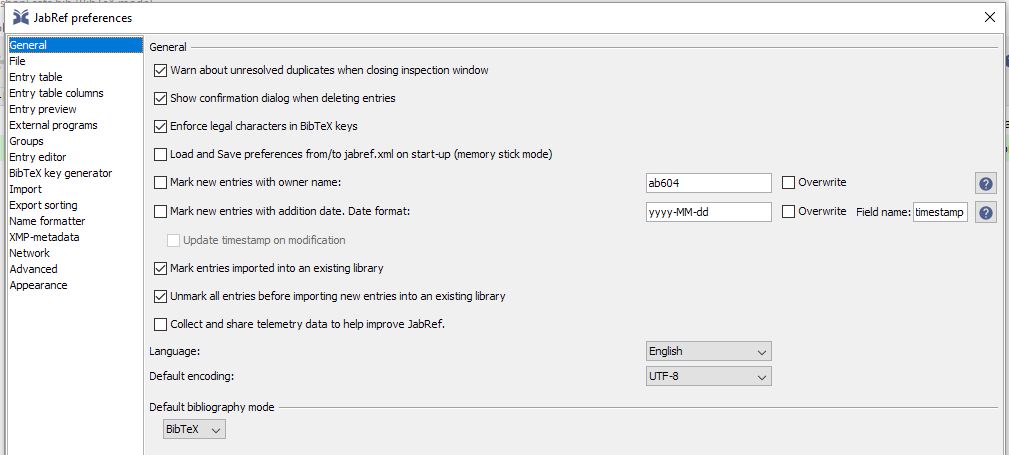1. Then go to `Options > Preferences > Bibtex key generator` and set this to `[auth:lower][year]` and then `OK`.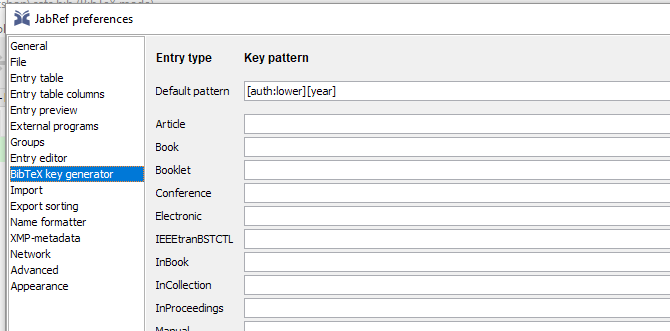1. Then create a library `File > New Bibtex Library`.

2. If you have exported your publications from EndNote or other software as bibtex you can import this into your new library and then highlight the list and click on the key icon to generate bibtex keys.

3. Alternatively, we can generate the list using the search function, here I entered a DOI and searched with Google Scholar: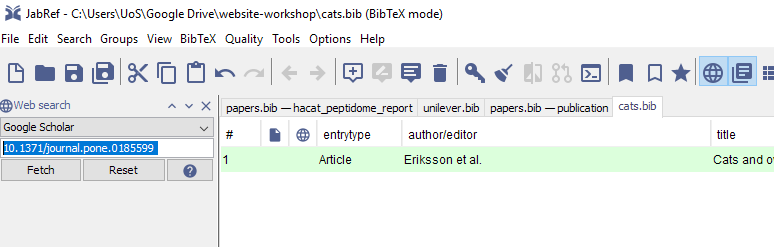Then I selected the publication and generated the key.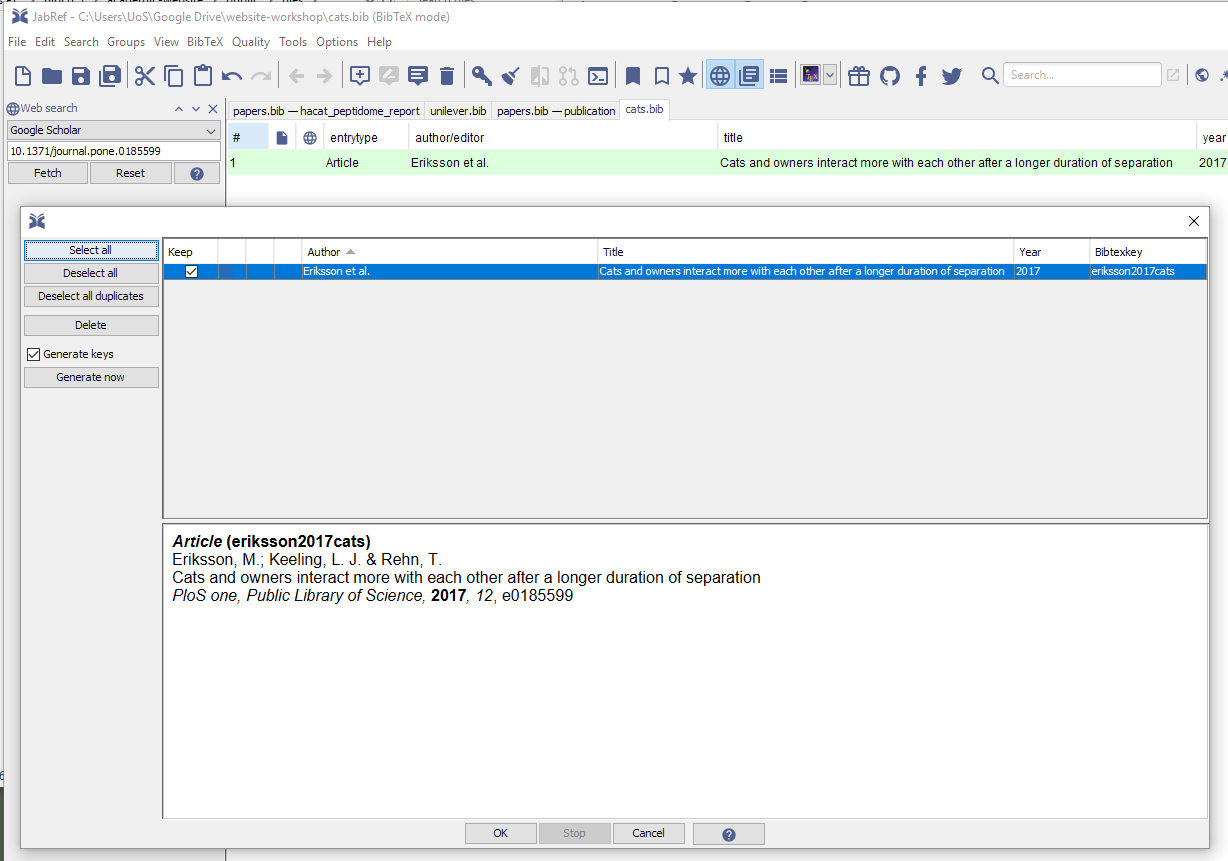1. Now we save the bibtex library. Here it is `cats.bib`. It’s just another text file so can be viewed in any text editor.

## 4.2 Converting Bibtex files

Converting to markdown requires some effort, fortunately Lorenzo Buesetto wrote a function that required a small hack to work with JabRef.

I found the function still may output files that need a bit of cleaning up, but generally it works really well.

We need the `tidyverse, RefManageR, anytime` packages installed.

``````bibtex_2academic <- function(bibfile,
outfold,
abstract = FALSE,
overwrite = FALSE) {
require(RefManageR)
require(dplyr)
require(stringr)
require(anytime)

# Import the bibtex file and convert to data.frame
mypubs   <-
.Encoding = "UTF-8") %>%
as.data.frame()

# assign "categories" to the different types of
# publications
mypubs   <- mypubs %>%
dplyr::mutate(
pubtype = dplyr::case_when(
bibtype == "Article" ~ "2",
bibtype == "Article in Press" ~ "2",
bibtype == "InProceedings" ~ "1",
bibtype == "Proceedings" ~ "1",
bibtype == "Conference" ~ "1",
bibtype == "Conference Paper" ~ "1",
bibtype == "MastersThesis" ~ "3",
bibtype == "PhdThesis" ~ "3",
bibtype == "Manual" ~ "4",
bibtype == "TechReport" ~ "4",
bibtype == "Book" ~ "5",
bibtype == "InCollection" ~ "6",
bibtype == "InBook" ~ "6",
bibtype == "Misc" ~ "0",
TRUE ~ "0"
)
)

# create a function which populates the md template
# based on the info
create_md <- function(x) {
# define a date and create filename by appending date
# and start of title
if (!is.na(x[["year"]])) {
x[["date"]] <- paste0(x[["year"]], "-01-01")
} else {
x[["date"]] <- "2999-01-01"
}

filename <- paste(
x[["date"]],
x[["title"]] %>%
str_replace_all(fixed(" "), "_") %>%
str_remove_all(fixed(":")) %>%
str_sub(1, 20) %>%
paste0(".md"),
sep = "_"
)
# start writing
if (!file.exists(file.path(outfold, filename)) |
overwrite) {
fileConn <- file.path(outfold, filename)
write("+++", fileConn)

# Title and date
write(paste0("title = \"", x[["title"]], "\""),
fileConn,
append = T)
write(paste0("date = \"", anydate(x[["date"]]), "\""),
fileConn,
append = T)

# Authors. Comma separated list, e.g. `["Bob Smith",
# "David Jones"]`.
auth_hugo <-
str_replace_all(x["author"], " and ", "\", \"")
auth_hugo <-
stringi::stri_trans_general(auth_hugo, "latin-ascii")
write(paste0("authors = [\"", auth_hugo, "\"]"),
fileConn,
append = T)

# Publication type. Legend:
# 0 = Uncategorized, 1 = Conference paper,
# 2 = Journal article
# 3 = Manuscript, 4 = Report, 5 = Book,  6 = Book
# section
write(paste0("publication_types = [\"", x[["pubtype"]],
"\"]"),
fileConn,
append = T)

# Publication details: journal, volume, issue,
# page numbers and doi link
publication <- x[["journal"]]
if (!is.na(x[["volume"]]))
publication <- paste0(publication,
", (", x[["volume"]], ")")
if (!is.na(x[["pages"]]))
publication <- paste0(publication,
", _pp. ", x[["pages"]], "_")
if (!is.na(x[["doi"]]))
publication <- paste0(publication,
", ",
paste0("https://doi.org/",
x[["doi"]]))

write(paste0("publication = \"", publication, "\""),
fileConn,
append = T)
write(paste0("publication_short = \"",
publication,
"\""),
fileConn,
append = T)

# Abstract and optional shortened version.
if (abstract) {
write(paste0("abstract = \"", x[["abstract"]], "\""),
fileConn,
append = T)
} else {
write("abstract = \"\"",
fileConn,
append = T)
}
write(paste0("abstract_short = \"", "\""),
fileConn,
append = T)

# other possible fields are kept empty. They can be
# customized later by
# editing the created md

write("image_preview = \"\"",
fileConn,
append = T)
write("selected = false", fileConn, append = T)
write("projects = []", fileConn, append = T)
write("tags = []", fileConn, append = T)
write("url_pdf = \"\"", fileConn, append = T)
write("url_preprint = \"\"",
fileConn,
append = T)
write("url_code = \"\"", fileConn, append = T)
write("url_dataset = \"\"",
fileConn,
append = T)
write("url_project = \"\"",
fileConn,
append = T)
write("url_slides = \"\"", fileConn, append = T)
write("url_video = \"\"", fileConn, append = T)
write("url_poster = \"\"", fileConn, append = T)
write("url_source = \"\"", fileConn, append = T)
#other stuff
write("math = true", fileConn, append = T)
write("highlight = true", fileConn, append = T)
# Featured image
write("image = \"\"", fileConn, append = T)
write("caption = \"\"", fileConn, append = T)

write("+++", fileConn, append = T)
}
}
# apply the "create_md" function over the
# publications list to generate
# the different "md" files.

apply(
mypubs,
FUN = function(x)
create_md(x),
MARGIN = 1
)
}``````

To use this function, save it as `bibtex_2academic.R` and then load the fcuntion into your R environment using `source("bibtex_2academic.R")`.

Then assuming you have a JabRef outputted Bixbtex file, here `cats.bib` we need to assign variables for the bibtex file and the output location, which in this case will be `content/publication`. Then we use these variables as arguement to the conversion function:

``````# Bibtex file in my directory
my_bibfile <- "cats.bib"
# Where I want the markdown output to go
outfold <- "content/publication"
# Use the conversion function
All being well, we should now have a markdown file for each publication (we only had one in this example) in the `content/publication`. It may need some manual tweaking if the format on the webpage isn’t quite right.